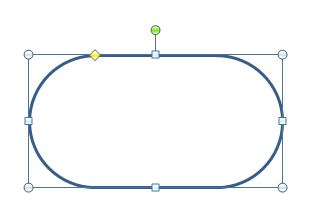# How to set and determine the rounding in AutoShapes with VBA

Published on
14,157 Points
7,957 Views
2 Endorsements
Approved
MS-Office allows to insert various shapes into  your documents (Word, Excel, Powerpoint, etc.), some of them have rounded angles, like

msoShapeRoundedRectangle,
msoShapeRound2DiagRectangle,
msoShapeRound2SameRectangle,
and others.

to adjust the rounding, you have normally to use the yellow diamond adjustment handle.

In VBA, you can use Adjustments. Item(1), mostly Item 1, sometimes Item 2 as well

For example in Excel:
``````Sub GetShapeRounding()

Dim myDocument As Worksheet
Dim oShape As Shape

Set myDocument = Worksheets(1)
Set oShape = myDocument.Shapes(1)

Set oShape = Nothing

End Sub

Sub SetShapeRounding()

Dim myDocument As Worksheet
Dim oShape As Shape

Set myDocument = Worksheets(1)
Set oShape = myDocument.Shapes(1)

Set oShape = Nothing

End Sub
``````
According to the microsoft online help (http://msdn.microsoft.com/en-us/library/office/bb265480(v=office.12).aspx), it is an adjustment value in proportion to the width of the shape: 1.0 corresponds to the width of the shape. The maximum value is 0.5, or half way across the shape. But this is not quite true, because the maximum rounding can only be made on the shorter pair of sides, creating a half-circle. 0 (zero) sets a no rounding to the shape.In this example, the adjustment measure is 0.5, which is half the height of the shape.

This adjustment method ensures that the rounding remains in proportion to the shape, when it is resized, but sometimes, we would like to have a determined rounding for a series of different-sized shapes.

Then you have to calculate the radius of the rounding to be able to reproduce it.

Like in this example :
``````Sub GetShapeRoundingRadius()

Dim myDocument As Worksheet
Dim oShape As Shape

Set myDocument = Worksheets(1)
Set oShape = myDocument.Shapes(1)

With oShape
If .Width < .Height Then
Else ' .Width >= .Height
End If
End With

Set oShape = Nothing

End Sub

Dim myDocument As Worksheet
Dim oShape As Shape

Set myDocument = Worksheets(1)
Set oShape = myDocument.Shapes(1)

With oShape
If .Width < .Height Then
Else ' .Width >= .Height
End If
End With

Set oShape = Nothing

End Sub
``````

Of course if the radius size is greater than half the side, then it will only round it until half-circle (min( Adjustments(1), 0.5)

You will see that due to rounding problems (again!) , that after having set the radius on the shape, if  you perform GetRadius, you will not have the same numbers, but the difference is really insubstantial.

In addition to this , we can now calculate the area of the shapes:

For example the rounded rectangle, the formula would be (height * width of the rectangle) less  4 * (radius^2) plus (pi * (radius^2))

simplified to (h * w) - ((4 - pi) * (r^2))

``````Sub GetShapeArea()

Dim myDocument As Worksheet
Dim oShape As Shape

sngPi = 3.141593

Set myDocument = Worksheets(1)
Set oShape = myDocument.Shapes(1)

With oShape
If .Width < .Height Then
Else ' .Width >= .Height
End If
sngArea = (.Width * .Height) - ((4 - sngPi) * (sngRadius * sngRadius))
End With

Debug.Print sngArea

Set oShape = Nothing

End Sub
``````
2